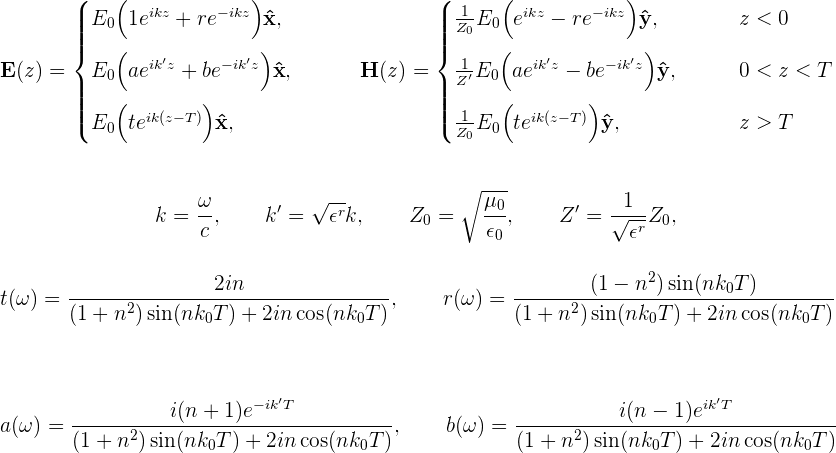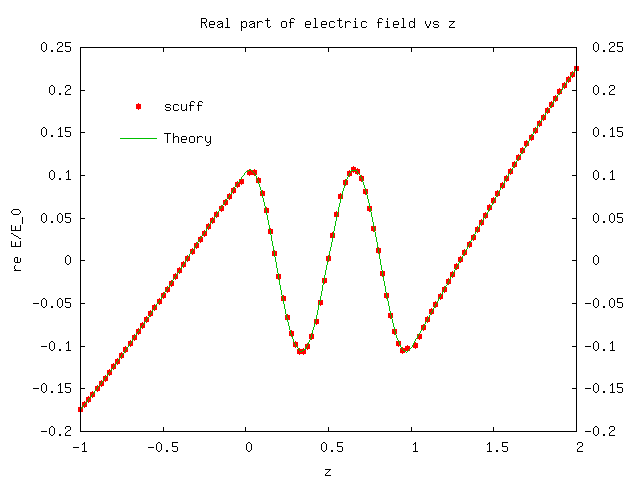Spatially-resolved study of plane-wave transmission through an (infinite-area) thin dielectric film

The previous examples dealt with compact scatterers. We'll next consider an extended geometry -- namely, a thin dielectric film of finite thickness in the z direction but infinite extent in the x and y directions. This is the same geometry for which we used scuff-transmission to look at the plane-wave transmission and reflection coefficients as a function of frequency in this example, but here we'll do a different calculation -- namely, we'll pick a single frequency and look at how the electric and magnetic fields vary in space, both inside and outside the thin film. (The files for this example may be found in the share/scuff-em/examples/ThinFilm directory of your scuff-em installation.)

The mesh file and .scuffgeo file for this geometry are discussed in the documentation for scuff-transmission. The geometry consists of a film of thickness T=1μm, with relative dielectric constant $\epsilon^r=100$, illuminated from below by a plane wave at normal incidence. (We'll take the incident field to be linearly polarized with E field pointing in the x direction.) The lower and upper surfaces of the film are at z=0 and z=T. For this geometry it is easy to solve Maxwell's equation directly to obtain the E and H fields directly at points below, within, and above the film:We will try to reproduce this behavior using scuff-scatter.

First create a little text file (ThinFilm.EvalPoints) containing the coordinates of a bunch of points on a straight line passing from below the film to above the film. Then put the following command-line arguments into a file called ThinFilm_58.args:

 geometry ThinFilm_58.scuffgeo
cache ThinFilm_58.scuffcache
omega 1.0
EPFile ThinFilm.EvalPoints
pwDirection 0 0 1
pwPolarization 1 0 0


and pipe it into scuff-scatter:

 scuff-scatter < scuff-scatter.args


This produces files named ThinFilm.scattered and ThinFilm.total. Looking at the first few lines of these files, we see that the 3rd data column on each line is the $z$ coordinate of the evaluation point, the 4th column is the real part of $E_x$, and the 12th column is the real part of $H_x$. Thus, plotting the 4th vs. 3rd and 12th vs. 3rd columns of the .total file yields plots of the total electric and magnetic field vs. $z$, whereupon we find good agreement with theory: